# How to Implement the XLOOKUP function in Alteryx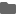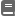2020.10.05

(日本語はこちらから)

## What is X-Lookup

In 2019, Microsoft© introduced XLOOKUP as a new function in excel. With this functionality, it is possible to find matched items in a table or a range, by rows. Thus it is possible to lookup for a term in one column and search that term to return its corresponding value in another column or another table. Few examples of XLOOKUP are discussed here and methods are provided for doing it in Alteryx.

1) Search & find: It is possible to search for a keyword of a table and get all its corresponding matched results from another table. Here employee information with an array of results is returned by searching for an employee ID.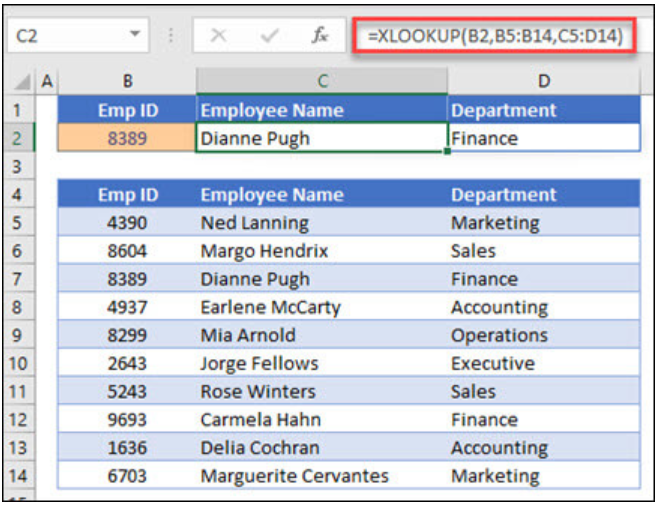2) Find the next larger (or smaller): It is possible to keyword an item from a table and get the adjoining value of its corresponding match in another table. Here, income entered in cell E2 is searched in the tax table for corresponding tax rate to return the next larger item.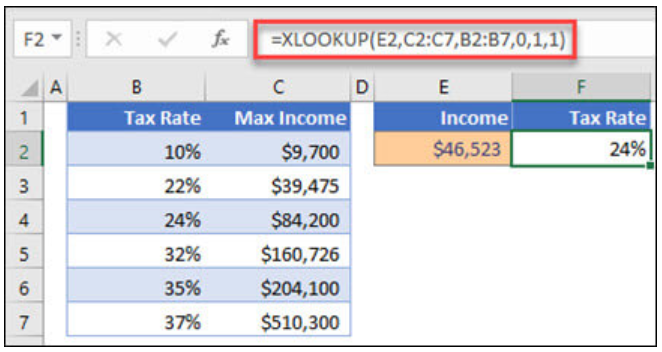3) Combine vertical and horizontal match: Using a nested loop of XLOOKUP, it is possible to combine a horizontal and vertical lookup to get a desired result. In this case, Gross Profit of column B and Qtr1 is searched in another table and the matched item at the intersection of the two is returned.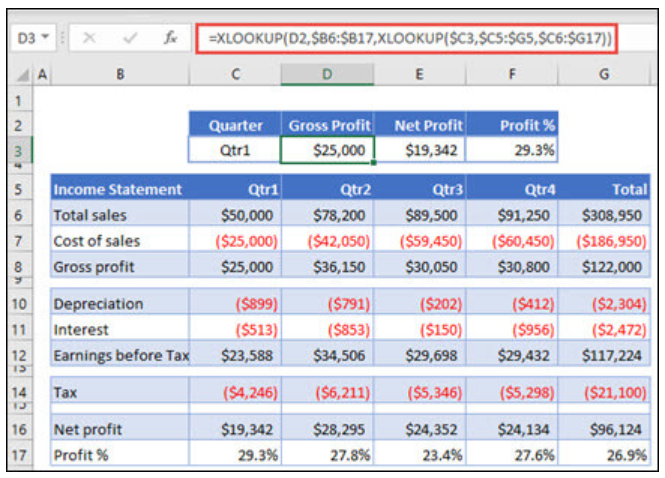4) Sum of values within a range: It is possible to feed the range of items from one table and get the resulting sum from an adjoining column of another table. Here, the SUM function, and two XLOOKUP functions nested together sums-up all the values between two ranges.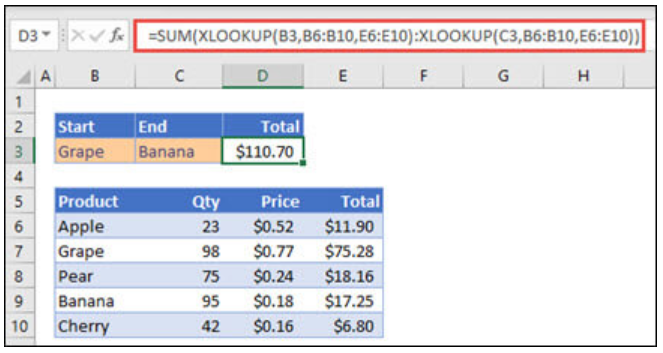## How to do it in Alteryx

There is no direct equivalent function of XLOOKUP in Alteryx, however by combining a few tools the desired results can be achieved.

1) Search & find: This can be achieved either by a “Filter” or “Join” tool or by appending fields in a “Find and Replace” tool whichever suits the scenario.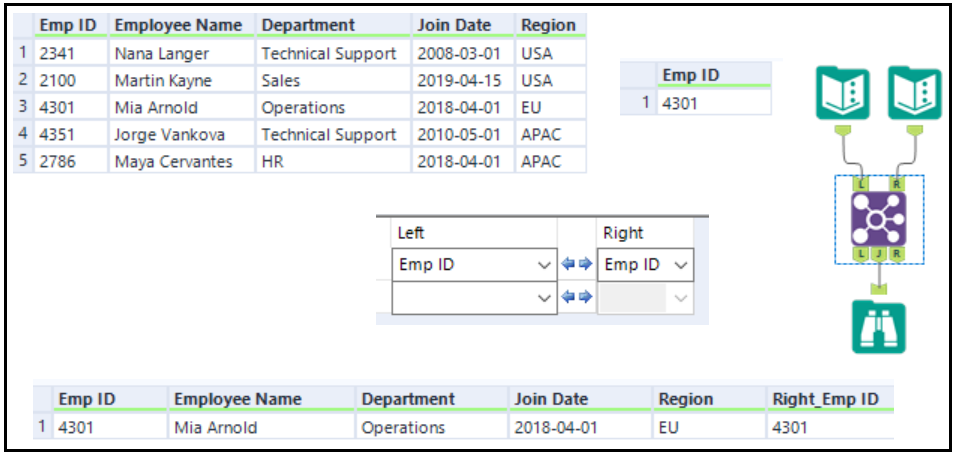2) Find the next larger (or smaller): There are several ways, but using the basic tools, this can be achieved with a combination of “Append Fields” and “Multi-row Formula” as shown below.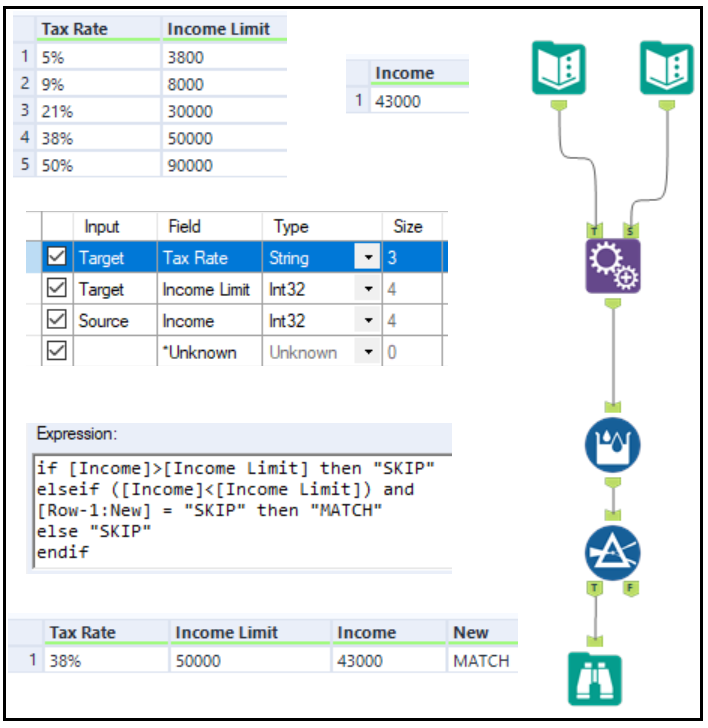3) Combine vertical and horizontal match: Using a “Join” tool or a combination of “Field Info” “Sample” and “Join” tool as shown here.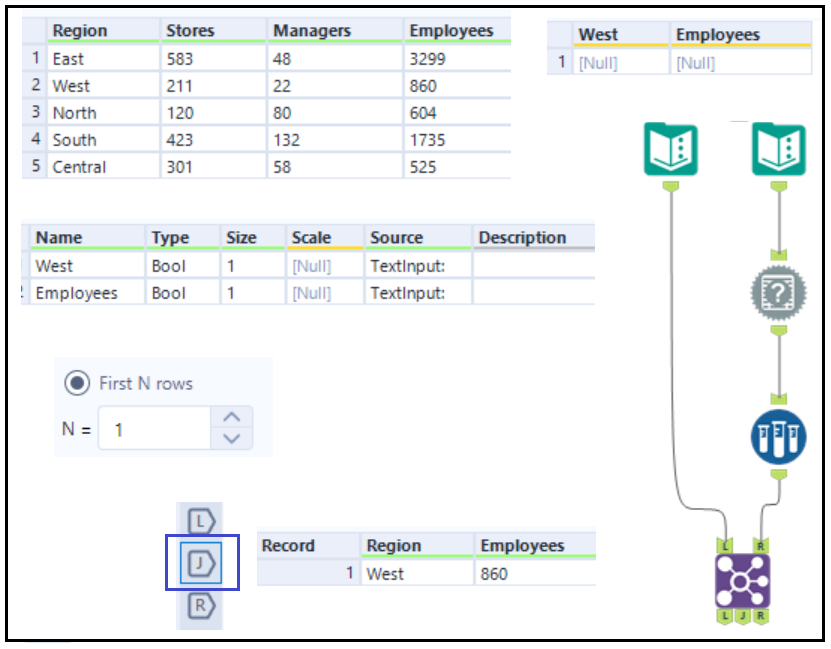4) Sum of values within a range: Lastly, another use of XLOOKUP as a nested loop can be achieved using Alteryx. To obtain the sum of values within a range you can use tools such as, “Multi Row Formula”, “Filter” and “Summarize” tools as shown below: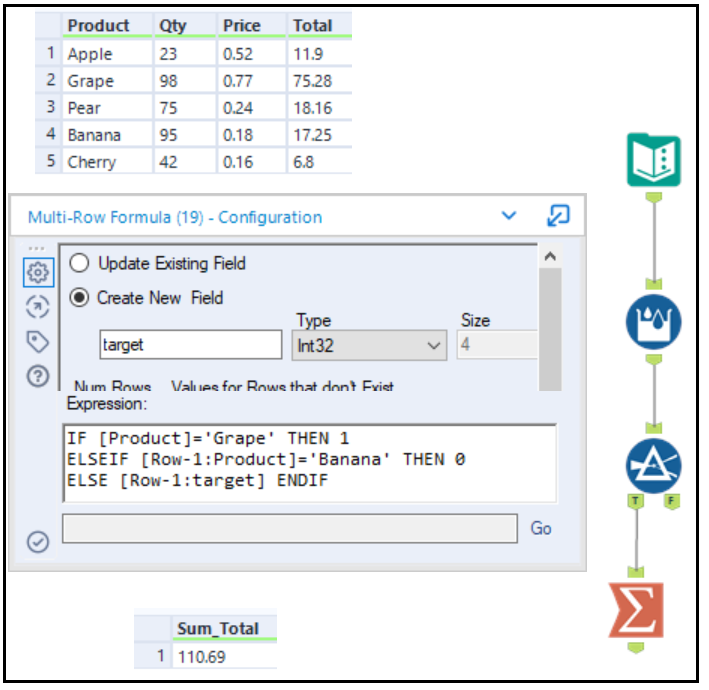## Conclusion

Although there is not a direct equivalent function of XLOOKUP in Alteryx, but by using a combination of basic tools, this functionality can be achieved easily.

### Alteryxの導入なら、クラスメソッドにおまかせください